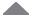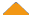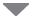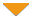Run Code  | Code Wall  | Users  | Misc  | Feedback  | About  | Login  | Theme  | Privacy

## Subset_sum_problem

 run  | edit  | history  | help0using namespace std; // dp[i][j] is going to store true if sum j is // possible with array elements from 0 to i. bool** dp; void display(const vector& v) { ``````for (int i = 0; i < v.size(); ++i) printf("%d ", v[i]); printf("\n"); `````` } // A recursive function to print all subsets with the // help of dp[][]. Vector p[] stores current subset. void printSubsetsRec(int arr[], int i, int sum, vector& p) { ``````// If we reached end and sum is non-zero. We print // p[] only if arr is equal to sun OR dp[sum] // is true. if (i == 0 && sum != 0 && dp[sum]) { p.push_back(arr[i]); display(p); return; } // If sum becomes 0 if (i == 0 && sum == 0) { display(p); return; } // If given sum can be achieved after ignoring // current element. if (dp[i-1][sum]) { // Create a new vector to store path vector b = p; printSubsetsRec(arr, i-1, sum, b); } // If given sum can be achieved after considering // current element. if (sum >= arr[i] && dp[i-1][sum-arr[i]]) { p.push_back(arr[i]); printSubsetsRec(arr, i-1, sum-arr[i], p); } `````` } // Prints all subsets of arr[0..n-1] with sum 0. void printAllSubsets(int arr[], int n, int sum) { ``````if (n == 0 || sum < 0) return; // Sum 0 can always be achieved with 0 elements dp = new bool*[n]; for (int i=0; i p; printSubsetsRec(arr, n-1, sum, p); `````` } // Driver code int main() { ``````int arr[] = {1, 2, 3, 4, 5}; int n = sizeof(arr)/sizeof(arr); int sum = 10; printAllSubsets(arr, n, sum); return 0; `````` }   by  hacker much, 14 days ago Please log in to post a comment.